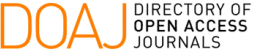### A New Expression for the Coulomb Potential Corresponding to the Product of Two Exponential Functions Based on the Properties of the Integral Representations of the Bessel Functions

C. J. Alturria Lanzardo, J. E. Pérez, M. L. Tardivo, G. Fraschetti, J. C. Cesco

#### Abstract

The calculation of the Coulomb Potential corresponding to the product of two Exponential Type Functions, inherently has numerical challenges that must be resolved. In order to address these problems, in this paper it is presented a new partition of the Coulomb Potential. The proposed partition involves two terms. One of the terms is a one-dimensional integral, which allows geometrical and statistical interpretations. The other term is proportional to a Modified Bessel Function and it is obtained from a two-step procedure. As a first step, a Non-Rational Function is used for approximating one of the two integrals involved. Then, the remaining improper integral can be identified with an integral representation of an appropriate Modified Bessel Function. The existence of such a Non-Rational Approximant is proved and its numerical performance is shown through some examples.

#### Keywords

Bessel functions; non-rational functions; integral representation; improper integrals; oscillating integrand; Exponential Type Functions; Coulomb Potential

PDF

#### References

J. E. Pérez, O. E. Taurian, A. Bouferguene, and P. E. Hoggan, “On a transformation for the electrostatic potential, generated by the product of two 1s Slater type orbitals, giving an efficient expression,” Advances in Quantum Chemistry, vol. 67, pp. 65–71, 2013.

C. J. Alturria. Lanzardo, J. Pérez, M. L. Tardivo, G. Fraschetti, and J. C. Cesco, “Potencial de coulomb para funciones 1s de Slater expresado en términos de funciones de Bessel,” in Matemática Aplicada Computacional e Industrial, vol. 8, pp. 81–84, ASAMACI, 2021.

I. Stegun and M. Abramowitz, Handbook of mathematical functions and tables, Bessel function of fractional order. National Bureau of Standards, App Math. Series, Dover, 1972.

J. C. Cesco, J. E. Pérez, C. C. Denner, G. O. Giubergia, and A. E. Rosso,

“Rational Approximants to Evaluate four-center Electron Repulsion ntegrals for 1s Hydrogen Slater Type Functions,” Applied Numerical Mathematics, vol. 55, pp. 179–190, 2005.

C. J. Alturria Lanzardo, J. Pérez, and J. Cesco, “Aproximación de integrales impropias cuyo integrando tiene una parte oscilante,” in Matemática Aplicada Computacional e Industrial, vol. 7, pp. 181–184, ASAMACI, 2019.

DOI: https://doi.org/10.5540/tcam.2022.024.01.00001

### Refbacks

• There are currently no refbacks.

Trends in Computational and Applied Mathematics

A publication of the Brazilian Society of Applied and Computational Mathematics (SBMAC)

Indexed in: